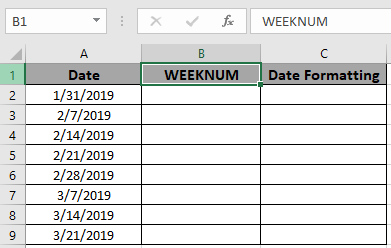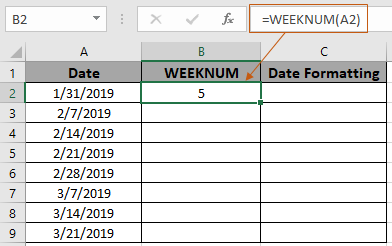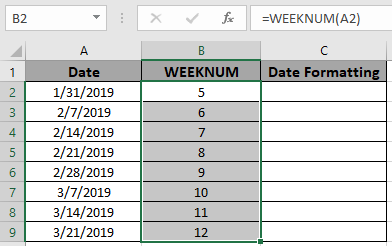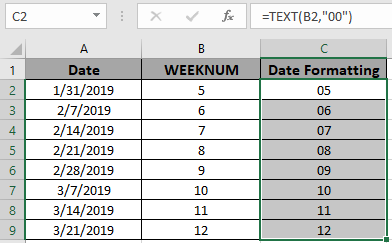# Zero padding to the week numbers in Excel

WEEKNUM function returns the week number of a year. There are 53 weeks in a year. So the function returns a value between 1 to 53.

As we already know, WEEKNUM function takes the date as an argument and returns its week number in the particular year.

Syntax:

= WEEKNUM ( Excel Date)

Here, we have some dates to test the function.Use the formula:

=WEEKNUM(A2)The 31st of the January month of the year returns 5.

Now copy the formula in the remaining cells using Shortcut key Ctrl + D.The function returned the week number of the year as required.

We now need to pad zero on the above results. So how do we do zero padding in Excel? The TEXT function!

Here TEXT function is used to change the cell value formatting.
Syntax

= TEXT ( text , text_format )

Now let's use the TEXT function on the results of the WEEKNUM function.

Use the formula:

= TEXT ( B2 , "00" )

"00" : 2 digit number. As we know that week number in year is never greater than 53.As you can see from the above snapshot we have added padding of zero before week number. When the week number is one digit, it comes with 1 zero padded in front of the number. When the week number is a two digit number, no padding of zero happens.

Hope it was explanatory enough and you now know how to pad zero with week numbers in Excel. Explore more articles on Excel Date & Time function here. Please feel free to state your query or feedback for the above article.

Related Articles

How to use the WEEKNUM function in Excel

How to use the TEXT function in Excel

Convert Text to Numbers

Popular Articles

The VLOOKUP Function in Excel

Conditional Formatting with formula 2016

create Overlay Chart in Excel 2016

Terms and Conditions of use

The applications/code on this site are distributed as is and without warranties or liability. In no event shall the owner of the copyrights, or the authors of the applications/code be liable for any loss of profit, any problems or any damage resulting from the use or evaluation of the applications/code.